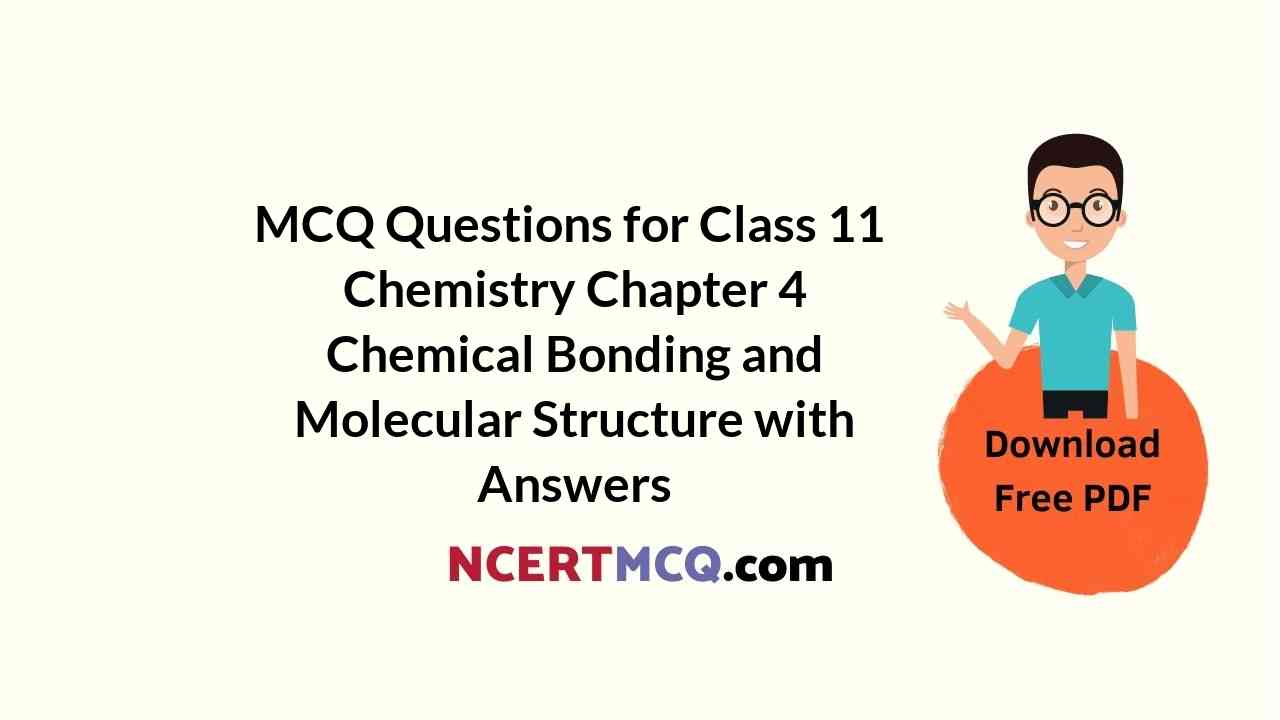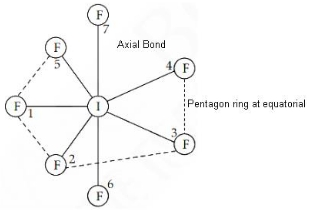Check the below NCERT MCQ Questions for Class 11 Chemistry Chapter 4 Chemical Bonding and Molecular Structure with Answers Pdf free download. MCQ Questions for Class 11 Chemistry with Answers were prepared based on the latest exam pattern. We have provided Chemical Bonding and Molecular Structure Class 11 Chemistry MCQs Questions with Answers to help students understand the concept very well.

## Chemical Bonding and Molecular Structure Class 11 MCQs Questions with Answers

Chemical Bonding Class 11 MCQ Question 1.
The bond length between hybridised carbon atom and other carbon atom is minimum in
(a) Propane
(b) Butane
(c) Propene
(d) Propyne

Explanation:
The C – C bond length = 1.54 Å, C = C bond length = 1.34 Å and C ≡ C bond length = 1.20 Å.
Since propyne has a triple bond, therefore it has minimum bond length.

Chemical Bonding And Molecular Structure MCQ Question 2.
The number of nodal planes present in s × s antibonding orbitals is
(a) 1
(b) 2
(c) 0
(d) 3

Explanation:
In an antibonding molecular orbital, most of the electron density is located away from the space between the nuclei, as a result of which there is a nodal plane (i.e, a plane at which the electron density is zero) between the nuclei.

Class 11 Chemistry Chapter 4 MCQ With Answers Question 3.
The hybrid state of sulphur in SO2 molecule is :
(a) sp²
(b) sp³
(c) sp
(d) sp³d

Explanation:
The hybridisation of sulphur in SO2 is sp². Sulphur atom has one lone pair of electrons and two bonding domains. Bond angle is <120° and molecular geometry is V-shape, bent or angular

Class 11 Chemistry Chapter 4 MCQ Question 4.
Which one of the following does not have sp² hybridised carbon?
(a) Acetone
(b) Acetic acid
(c) Acetonitrile
(d) Acetamide

Explanation:
Acetonitrile does not contain sp² hybridized carbon.

MCQ Questions For Class 11 Chemistry Chapter 4 Question 5.
Which of the following will have the lowest boiling point?
(a) 2-MethylButane
(b) 2-MethylPropane
(c) 2,2-Dimethylpropane
(d) n-Pentane

Explanation:
Boiling point increases with increase in molecular mass. For the compounds with the same molecular mass, boiling point decreases with an increase in branching.
The molecular mass of 2-Methylbutane: 72 g mol-1
The molecular mass of 2-Methylpropane: 58 g mol-1
The molecular mass of 2, 2-Dimethylpropane: 72 g mol-1
The molecular mass of 2-Methylbutane: 72 g mol-1
2-Methylpropane has the lowest molecular mass among all of the given compounds.
Thus, 2-Methylpropane has the lowest boiling point among the given options.

My question is that how to calculate bond order for open and closed sheel.

Chemical Bonding And Molecular Structure Class 11 MCQ Question 6.
Among the following the maximum covalent character is shown by the compound
(a) MgCl2
(b) FeCl2
(c) SnCl2
(d) AlCl3

Explanation:
We know that, extent of polarisation ∝ covalent character in ionic bond. Fajans rule states that the polarising power of cation increases, with increase in magnitude of positive charge on the cation Therefore, polarising power ∝ charge of cation.

The polarising power of cation increases with the decrease in the size of a cation. Therefore, polarising (power) ∝ (1)/ (size of cation)
Here the AlCl3 is satisfying the above two conditions i.e., Al is in +3 oxidation state and also has small size. So it has more covalent character.

MCQ Of Chemical Bonding Class 11 Question 7.
Among the following mixtures, dipole-dipole as the major interaction, is present in
(a) benzene and ethanol
(b) acetonitrile and acetone
(c) KCl and water
(d) benzene and carbon tetrachloride

Explanation:
Dipole-dipole interactions occur among the polar molecules. Polar molecules have permanent dipoles. The positive pole of one molecule is thus attracted by the negative pole of the other molecule. The magnitude of dipole-dipole forces in different polar molecules is predicted on the basis of the polarity of the molecules, which in turn depends upon the electro negativities of the atoms present in the molecule and the geometry of the molecule (in case of polyatomic molecules, containing more than two atoms in a molecule).

Ch 4 Chemistry Class 11 MCQ Question 8.
The value of n in the molecular formula BenAl2Si6O18 is
(a) 3
(b) 5
(c) 7
(d) 9

Explanation:
BenAl2Si6O18
The oxidation states of each element
Be = +2
Al = +3
Si = +4
O = -2
(2n) + (3 × 2) + (4 + 6) + (−2 × 18) = 0
or 2n + 30 − 36 = 0
or 2n = 6
or n = 3

MCQ On Chemical Bonding Class 11 Pdf Question 9.
Which of the following types of hybridisation leads to three dimensional geometry of bonds around the carbon atom?
(a) sp
(b) sp²
(c) sp³
(d) None of these

Explanation:
sp² hybrid structures have trigonal planar geometry, which is two dimensional.

Chemical Bonding MCQ Class 11 Question 10.
An atom of an element A has three electrons in its outermost orbit and that of B has six electrons in its outermost orbit. The formula of the compound between these two will be
(a) A3B6
(b) A2B3
(c) A3B2
(d) A2B

Explanation:
A has 3 electrons in outermost orbit and B has 6 electrons in its outermost orbits. So A can give three electrons to complete its octet and B needs 2 electrons to complete its octet. So 2 atoms of A will release 6 electrons and 3 atoms of B will need six electrons to complete their octet
So, the formula will be A2​B3

Class 11 Chemistry Ch 4 MCQ Question 11.
The maximum number of hydrogen bonds that a molecule of water can have is
(a) 1
(b) 2
(c) 3
(d) 4

Explanation:
Each water molecule can form a maximum of four hydrogen bonds with neighboring water molecules. The two hydrogens of the water molecule can form hydrogen bonds with other oxygens in ice, and the two lone pair of electrons on oxygen of the water molecule can attract other hydrogens in ice. Hence, 4 possible hydrogen bonds.

MCQ Questions On Chemical Bonding Class 11 Question 12.
The number of types of bonds between two carbon atoms in calcium carbide is
(a) Two sigma, two pi
(b) One sigma, two pi
(c) One sigma, one pi
(d) Two sigma, one pi

Answer: (b) One sigma, two pi
Explanation:
A single bond between two atoms is always considered as sigma bond.
A double bond between two atoms is always considered as one sigma and one pi bond
A triple bond between two atoms is always considered as one sigma bond and two pi bonds.
So according to the given structure CaC2 (Calcium carbide) has 1 sigma and 2 pi bonds

Class 11 Chemical Bonding MCQ Question 13.
Based on lattice enthalpy and other considerations which one the following alkali metals chlorides is expected to have the higher melting point?
(a) RbCl
(b) KCl
(c) NaCl
(d) LiCl

Explanation:
The highest melting point will be NaCl, it is because, the lattice energy decreases as the size of alkali metal increases so going down the group the melting point decreases, but due to the covalent bonding in LiCl, its melting point is lower than NaCl and so NaCl is expected to have maximum melting point in the alkali chlorides.​

MCQ Of Chapter 4 Chemistry Class 11 Question 14.
Dipole-induced dipole interactions are present in which of the following pairs?
(a) H2O and alcohol
(b) Cl2 and CCl4
(c) HCl and He atoms
(d) SiF4 and He atoms

Answer: (c) HCl and He atoms
Explanation:
HCl is polar (μ ≠ 0) and He is non-polar (μ = 0) gives dipole-induced dipole interaction.

Chemistry Class 11 Chapter 4 MCQ Question 15.
Among the following mixtures, dipole-dipole as the major interaction, is present in
(a) benzene and ethanol
(b) acetonitrile and acetone
(c) KCl and water
(d) benzene and carbon tetrachloride

Explanation:
Dipole-dipole interactions occur among the polar molecules. Polar molecules have permanent dipoles. The positive pole of one molecule is thus attracted by the negative pole of the other molecule. The magnitude of dipole-dipole forces in different polar molecules is predicted on the basis of the polarity of the molecules, which in turn depends upon the electro negativities of the atoms present in the molecule and the geometry of the molecule (in case of polyatomic molecules, containing more than two atoms in a molecule).

Chapter 4 Chemistry Class 11 MCQ Question 16.
The charge/size ratio of a cation determines its polarizing power. Which one of the following sequences represents the increasing order of the polarizing order of the polarizing power of the cationic species, K+, Ca++, Mg2+, Be2+?
(a) Ca2+ < Mg2+ < Be+ < K+
(b) Mg2+ < Be2+ < K+ < Ca2+
(c) Be2+ < K+ < Ca2+ < Mg2+
(d) K+ < Ca2+ < Mg2+ < Be2+

Answer: (d) K+ < Ca2+ < Mg2+ < Be2+
Explanation:
High charge and small size of the cations increases polarisation.
As the size of the given cations decreases as
K+ > Ca2+ > Mg2+ > Be2+
Hence, polarising power decreases as K+ < Ca2+ < Mg2+ < Be2+

Chapter 4 Chemistry Class 11 MCQs Question 17.
The species having pyramidal shape is
(a) SO3
(b) BrF3
(c) SiO32-
(d) OSF2

Explanation:
The species having a pyramidal shape according to VSEPR theory is OSF2. The central S atom has 3 bonding domains (one S = O double bond and two S−F single bonds) and one lone pair of electrons.

The electron pair geometry is tetrahedral and molecular geometry is pyramidal. This is similar to the ammonia molecule.

MCQ Chemical Bonding Class 11 Question 18.
The structure of IF7 is
(a) Pentagonal bipyramid
(b) Square pyramid
(c) Trigonal bipyramid
(d) Octahedral

Explanation:
IF7 Hybridization is sp³d³
Structure is Pentagonal bipyramidal.MCQ On Chemical Bonding Class 11 Question 19.
The outer orbitals of C in ethene molecule can be considered to be hybridized to give three equivalent sp² orbitals. The total number of sigma (s) and pi (p) bonds in ethene molecule is
(a) 1 sigma (s) and 2 pi (p) bonds
(b) 3 sigma (s) and 2 pi (p) bonds
(c) 4 sigma (s) and 1 pi (p) bonds
(d) 5 sigma (s) and 1 pi (p) bonds

Answer: (d) 5 sigma (s) and 1 pi (p) bonds
Explanation:
According to valence bond theory, two atoms form a covalent bond through the overlap of individual half-filled valence atomic orbitals, each containing one unpaired electron. In ethene, each hydrogen atom has one unpaired electron and each carbon is sp² hybridized with one electron each sp² orbital. The fourth electron is in the p orbital that will form the pi bond. The bond order for ethene is simply the number of bonds between each atom: the carbon-carbon bond has a bond order of two, and each carbon-hydrogen bond has a bond order of one.

MCQ On Chemical Bonding With Answers Pdf Question 20.
Which of the following is a linear molecule?
(a) ClO2
(b) CO2
(c) NO2
(d) SO2